## Surfaces

A parametric surface is described by a map of a rectangle in (u,v)-space into (x,y,z)-space. The rectangle is the product of the intervals [uMin,uMax] and [vMin,vMax]. Each point (u,v) of the rectangle is mapped to a three-dimensional point P with coordinates (x(u,v), y(u,v), z(u,v)). The mapping is given by writing Pascal procedures that defines the values of x, y, and z as functions of u,v and nine global "parameters", called aa, bb, cc, dd, ee, ff, gg, hh, ii. Most surfaces need only 1, 2, 3 or even none of these parameters. (For example, an ellipsoid depends on three semi-axes, aa,bb,cc).

To discretize the surface, the domain in (u,v) space is divided into a grid which has uResolution equi-spaced points in the u-direction running from uMin to uMax, and and vResolution equi-spaced points in the v-direction running from vMin to vMax. This grid divides the (u,v) domain rectangle into subrectangles. When a surface is "Create"-ed, the mapping (u,v) ---> (x,y,z) is applied to each of the grid points, so each of the subrectangles in (u,v)-space is mapped to a quadrilateral in (x,y,z)-space called a "patch". This collection of patches is what actually represents the surface immersed in 3-dimensional space.

To "see" the surface, it is necessary to make a 2-dimensional projection of these patches on the screen. The program does this by projecting each patch onto a plane (called ImagePlane) that is at a distance (called FocalLength) from ViewPoint" and is orthogonal to a direction called "ViewDirection". The point where the line from ViewPoint in the direction ViewDirection meets the ImagePlane is called "ImagePlaneCenter" and is always placed at the center of the Graphics Window. There is another distance, called ClipDistance, usually set to a fraction (ClipRatio) of FocalLength, and a patch is "clipped" (i.e., not projected) if any of its vertices lie behind the ClipPlane (the plane parallel to the Image Plane at a distance ClipDistance from the ViewPoint). The projection onto ImagePlane can be either a perspective projection or it can be orthographic, and the choice between these options is made in the View menu, Of course, a perspective projection has its center of projection at ViewPoint, meaning that a point p is projected onto the point q of ImagePlane where the line from ViewPoint to p meets ImagePlane. In the orthographic case, the point q is just the orthogonal projection of p on ImagePlane.

One more piece of data is needed to render a patch on the computer screen, namely the relation between distances in the ImagePlane and distances on the monitor. This is defined by an integer called "Scale", which gives the number of pixels per unit length on ImagePlane---i.e., on a "standard" monitor, with 72 dpi resolution, a line segment of unit length in ImagePlane will have length Scale/72 inches on the monitor. (The "normal" value of Scale is chosen to be one-tenth the sum of the height and width of the Graphics Window in pixels. This has the effect of scaling objects proportionally to the window size, and usually gives a value of scale between 70 and 100.)The patches are sorted by the distance of their centers to the ViewPoint, and are drawn on the screen in order of farthest to nearest ("Painter's Algorithm").

The patches are illuminated by a "light" shining in the direction ViewDirection and the brightness of a patch depends on the cosine of the angle the normal to the patch makes with ViewDirection. (It is also possible to "color" the surface and to shine colored lights on it from several different sources. For a discussion of the details see Color.)

For some purposes it may be better to make the patches "transparent" and view the surface as if it were made of chicken wire. To switch back and forth, choose either "Wire Frame" or "Patch" from the View menu.

If you click and drag a surface, it rotates inside the virtual sphere. If you depress the Command key, then when you drag the surface will follow the mouse, and if you depress the Shift key and move the cursor up or down then the surface gets smaller or larger.)

When you have selected a particular surface from the Surface menu, a version with certain default parameter values will be displayed. You can then choose "About this Object'' from the Action Menu to see how the surface depends on these parameters. You can change the values of these parameters by choosing Set Parameters... in the Settings Menu before re-creating the surface.

You can define a parametric surface in cartesian, polar, or cylindrical by choosing User Defined (Cartesian)... etc. from the Surfaces menu. This brings up a dialog that will permit a user to create three expressions (e.g., for P.x, P.y, and P.z ) involving the two surface variables u and v and the nine parameters, aa,bb, ... , ii. There is some checking done to see that these are well-formed formulas, but you should be careful to avoid other errors (such as an implicit division by zero) that could have unpredictable consequences. If you mess up badly in editing the expressions, press the Cancel button and then choose User Defined... again to start over. When you have finished editing, either press the Create button to immediately create the surface, or press the OK button and make various choices from the Settings menu before choosing Create from the Surfaces menu. Note that if you want to create the graph of a function, f(x,y), i.e., display the surface z=f(x,y), then you can use the parametric equations x=u, y=v, and z=f(u,v). Similarly, choosing User Defined (Rotation)... will bring up a dialog that will permit a user to define a parametric curve in the x,y-plane as a function of a curve parameter u, and that curve will then be rotated about the y-axis to produce a surface of rotation. See the documentation on User Defined Objects for details of how to enter formulas in these dialogs.

After a parametric surface is created, the Show Parallel Surfaces menu item of the Action menu will become enabled. Choosing it will first show the two focal sets of the surface and then start an animation showing the parallel surfaces to the given surfaces (i.e., the surfaces at a constant distance out along the surface normal). If you hold down the Shift key during the animation you will be able to see the focal sets until you release it (the parallel surfaces develop singularities where they meet the focal sets). Each press of the right-arrow key will speed up the animation, while each press of the left-arrow key will slow it down. Pressing the up-arrow key reverses the direction of the animation.

## Anand-Ward Solitons Christopher Anand

This subcategory was created to investigate the properties of solutions to an integrable Chiral model put forward by Richard Ward.

Very briefly, a Chiral Model is a physical model for fundamental forces in physics. It is a field theory, in that there are no particles in the sense of Newtonian mechanics, but fields taking values in a group. The classical solutions of the model are defined either as critical points of an energy functional, or directly as solutions of a differential equation. The equations are closely related to the harmonic map equations in differential geometry, and since harmonic maps are generalisations of minimal surfaces (i.e., soap films), this provides some insight into possible physical properties of the theory.

Chiral models are known to admit soliton solutions, which in the physical sense means that the energy densities of solutions are reminicent of configurations of particles: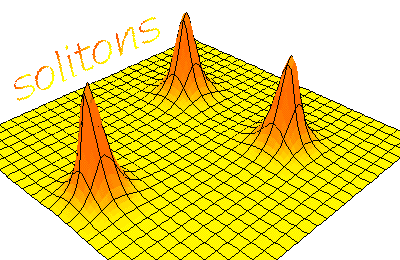(when graphed as a positive real-valued function of two variables); and they maintain this lumpy appearance as they evolve, with the possible changes in the configuration interpreted as an interaction of particles..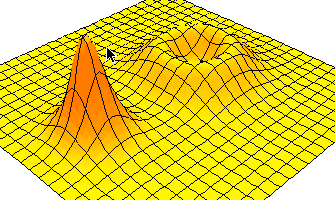The model considered here is a 2+1 dimensional SU(N) model, which means that the field is a map from R3 to the special unitary group, satisfying a differential equation whose symbol (leading order terms) is indefinite. In contrast, classical solutions to a gauge theory can be thought of as maps into a Lie algebra. Ward's equations are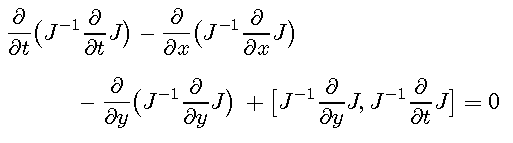The first three terms constitute the standard Chiral Model, to which Ward has added lower-order (torsion) terms. The lower order terms destroy the Lorentz invariance of the equations, but result in completely integrable equations. Completely integrable systems arose in Hamiltonian mechanics where they were defined to be systems possessing a complete set of integrals. From the point of view of differential geometry, the most practical definition is that the equations can be rewritten by defining a connection (or parametrised family of connections) for which the equation is the the condition that the curvature of the connection is zero. Solutions of the equations then correspond to flat connections, and the fact that flat connections have covariant constant gauges locally gives a natural method of integrating the equations.

These ideas are not new, but applications to new systems are of considerable importance. It is a matter of current interest to understand better the extent to which the theory of completely integrable systems can be understood within the Twistor Theory developed to study Yang-Mills gauge theory (see [L. Mason and N. Woodhouse, Integrability, Self-duality and Twistor Theory, OUP 1996]).

In this case, the mini-Twistor theory of magnetic monopoles [N. J. Hitchin, Monopoles and Geodesics, Commun. Math. Phys. 83 (1982), 579--602] (which is subsumed in the Yang-mills picture) can be used. The moral of the story is that after imposing the boundary condition that the solutions extend analytically to RP3 (where we view R3 as an open cell in real projective space) the general solution is of the form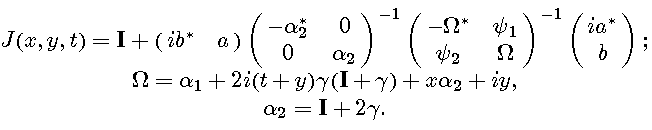It follows that the space of solutions has infinitely many finite-dimensional components, indexed by k, the size of the constant matrices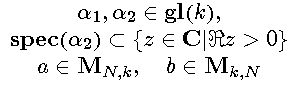appearing in the closed form. Because the static solutions correspond to harmonic spheres in SU(N), it is known that the component number (the size of the matrix alpha) is the energy of the static solutions in this component.

Ward's equations now become the matrix equation and the solutions will be smooth if the data satisfy the nondegeneracy conditions Data satisfying these conditions parametrise the space of solutions uniquely up to the action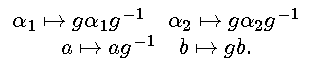of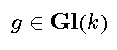Having discovered that the moduli space of solutions is (locally) finite dimensional, we would like to know how the geometry of this space is related to the geometry of the solutions. In particular, whether parameters (coordinates on the moduli space) can be found which predict the interactions of the solitons we see when viewing energy densities.

This is where you come in. By choosing 2x2, 3x3 or 4x4 monad data, from the AW Solitons submenu, you can enter your own parameters for solutions in the first three components of the moduli space of SU(3) solutions. You can then set the time using the Set Parameters dialogue (time is parameter aa ) and view the energy density of your solution. You can also change the horizontal scale (bb) vertical scale (cb) and cut off (dd) above which value, the energy will be replaced with the value (ee). The last feature is included because extremely concentrated energy-densities can produce puzzling displays.

All of the usual display controls can be used to view the surface. In particular Morphing can be used to create a moving picture of interacting solitons. (You will probably want to hold all of bb, cc, dd, and ee constant and vary aa only.)

Of course, you may want to start by viewing the pre-programmed solutions first.

Any questions about the use of the subcategory, this documentation, the geometry behind the construction of the solutions, or interpretations of the parameters in terms of the behaviour of the solutions should be directed to me!